Common Core: S.IC.5

# How to Find a P-Value from a T-Test Statistic

Author: Sophia Tutorial
##### Description:

Calculate a p-value for a left-tailed, right-tailed, or two-sided t-test with a given t-test statistic.

(more)

Sophia’s self-paced online courses are a great way to save time and money as you earn credits eligible for transfer to many different colleges and universities.*

No credit card required

37 Sophia partners guarantee credit transfer.

299 Institutions have accepted or given pre-approval for credit transfer.

* The American Council on Education's College Credit Recommendation Service (ACE Credit®) has evaluated and recommended college credit for 32 of Sophia’s online courses. Many different colleges and universities consider ACE CREDIT recommendations in determining the applicability to their course and degree programs.

Tutorial
what's covered
In this tutorial, you will learn how to find a p-value from a t-test statistic using the calculator, the t-table table, and Excel. Our discussion breaks down as follows:

1. Right-Tailed Test
1. Graphing Calculator
2. T-Table
3. Excel
2. Two-Tailed Test
1. Graphing Calculator
2. T-Table
3. Excel

## 1. Right-Tailed Test

The average ACT score in Illinois is a 20.9. There's one high school in particular that believes its students scored significantly better than the state average. Because the school believes that its students performed better, this is an upper tail test.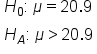In order to test this hypothesis, the school took a random sample of 15 students' scores and got an average of 22.5, with a standard deviation of 2.3.

Let's first ask ourselves which type of data we are dealing with. In this case, we're dealing with quantitative data, and we do not know the population standard deviation. We just know the sample standard deviation, so we're going to do a t-test.

The first step is to calculate the t-statistic:This gives us a t-score of 2.694. This score is plotted on the t-distribution below.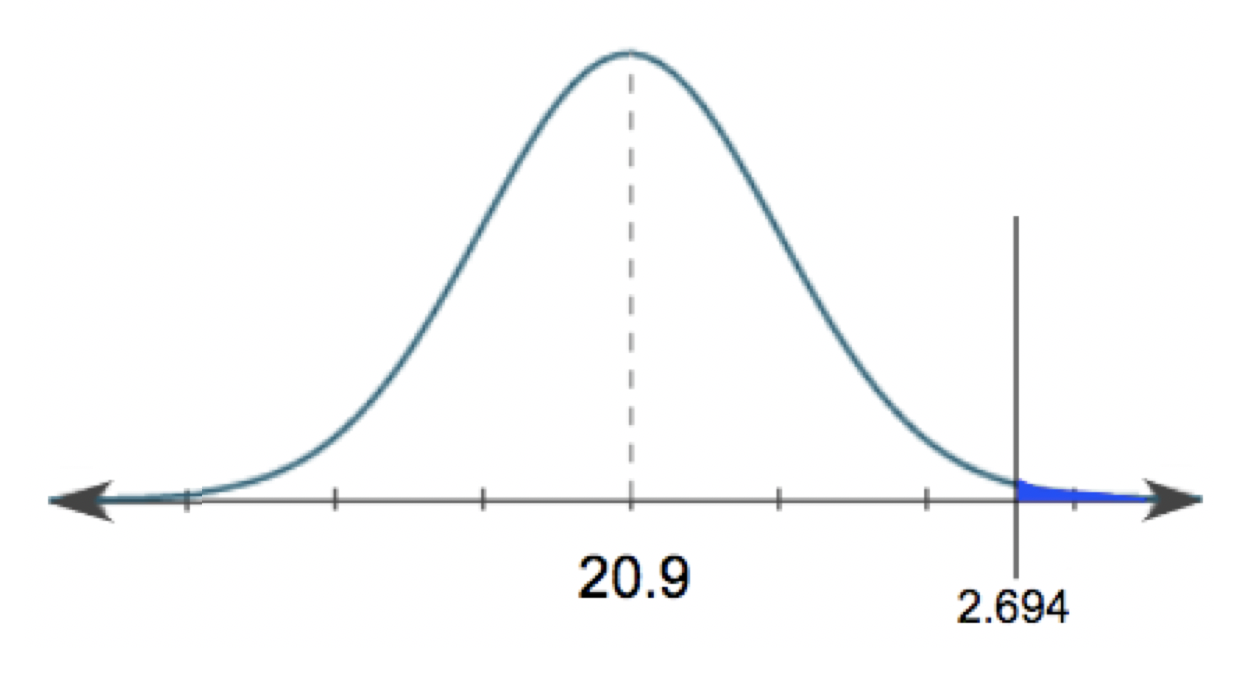1a. Graphing Calculator

In order to convert that into a p-value, the first method is on a graphing calculator. On your calculator, go ahead and hit 2nd DIST. We're interested in this sixth function, tcdf, which stands for "t cumulative density function."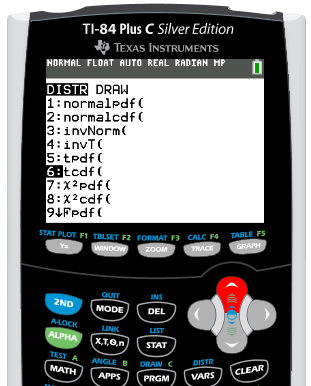When we're entering in the values for a "tcdf," it is always going to be the lower boundary of the shaded region, the upper boundary of the shaded region, then the degrees of freedom. Remember, the shape of this distribution changes with the sample size. In this case, the lower boundary of our shaded region is 2.694. The upper boundary is the top portion of our distribution, which is positive infinity. In order to indicate positive infinity to our calculator, we just enter a positive 99. Our degrees of freedom will be 14 because the degrees of freedom is sample size minus one, or 15 minus 1.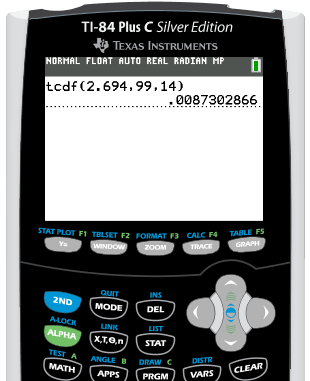For this particular problem, we have a p-value of 0.0087, or 0.87%.

1b. T-Table

We can also use the t-table. Now, tables sometimes can only get us an estimated p-value. They can't get us an exact p-value, like the calculator or Excel. But sometimes it's all we have, and it's definitely sufficient. In this case, remember we had a t-test statistic of 2.694. All of the values inside our t-table are a bunch of t-scores. On the left hand column, these are the degrees of freedom, and the top row contains all of our corresponding p-values.

What we're going to do is look for the corresponding degrees of freedom for our hypothesis test. In this case we had 14 degrees of freedom. In that same row, we're going to look for the closest thing possible to the t-score of 2.694.

t-Distribution Critical Values
Tail Probability, p
One-tail 0.25 0.20 0.15 0.10 0.05 0.025 0.02 0.01 0.005 0.0025 0.001 0.0005
Two-tail 0.50 0.40 0.30 0.20 0.10 0.05 0.04 0.02 0.01 0.005 0.002 0.001
df
1 1.000 1.376 1.963 3.078 6.314 12.71 15.89 31.82 63.66 127.3 318.3 636.6
2 0.816 1.080 1.386 1.886 2.920 4.303 4.849 6.965 9.925 14.09 22.33 31.60
3 0.765 0.978 1.250 1.638 2.353 3.182 3.482 4.541 5.841 7.453 10.21 12.92
4 0.741 0.941 1.190 1.533 2.132 2.776 2.999 3.747 4.604 5.598 7.173 8.610
5 0.727 0.920 1.156 1.476 2.015 2.571 2.757 3.365 4.032 4.773 5.893 6.869
6 0.718 0.906 1.134 1.440 1.943 2.447 2.612 3.143 3.707 4.317 5.208 5.959
7 0.711 0.896 1.119 1.415 1.895 2.365 2.517 2.998 3.499 4.029 4.785 5.408
8 0.706 0.889 1.108 1.397 1.860 2.306 2.449 2.896 3.355 3.833 4.501 5.041
9 0.703 0.883 1.100 1.383 1.833 2.262 2.398 2.821 3.250 3.690 4.297 4.781
10 0.700 0.879 1.093 1.372 1.812 2.228 2.359 2.764 3.169 3.581 4.144 4.587
11 0.697 0.876 1.088 1.363 1.796 2.201 2.328 2.718 3.106 3.497 4.025 4.437
12 0.695 0.873 1.083 1.356 1.782 2.179 2.303 2.681 3.055 3.428 3.930 4.318
13 0.694 0.870 1.079 1.350 1.771 2.160 2.282 2.650 3.012 3.372 3.852 4.221
14 0.692 0.868 1.076 1.345 1.761 2.145 2.264 2.624 2.977 3.326 3.787 4.140
15 0.691 0.866 1.074 1.341 1.753 2.131 2.249 2.602 2.947 3.286 3.733 4.073

Now, 2.694 falls somewhere in between these two values, 2.624 and 2.977. These t-scores correspond to the two p-values, 0.01 and 0.005, or 1% and 0.5%. Since it falls somewhere in the middle, we are actually just going to take the average of these two p-values.To do this, take 1% plus 0.5%; divide that by 2, and we get an estimated p-value of 0.0075, or 0.75% when using the t-table. It is not exactly the same as the value we found using the calculator; however, it is very close.

big idea
The t-table only shows positive values, however, you can still use this same method for a left-tailed test; you would just look for the positive of your t-statistic.

1c. Excel
To convert our t-test statistic into a p-value using Excel, go under the Formulas tab. We are going to insert a formula that falls under the Statistical column. We are looking for t-distribution dot rt, or T.DIST.RT, because we're performing a right-tail test.

Notice how there is no T.DIST.LT. If we're performing a left-tail test, we would just use the T.DIST. But since we're performing an upper-tail test, we are going to use T.DIST.RT. The first thing we are going to put in is the t-score, which was a positive 2.694, then 14 degrees of freedom. Hit Enter.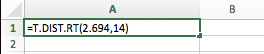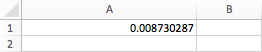Notice how we get the same p-value of 0.0087, or 0.87%, as when we used our calculator.

## 2. Two-Tailed Test

The quality assurance of a soda company wants to make sure that its machines are working in filling its 12-ounce cans of soda. Because it would be a problem if the machines were significantly over-filling and under-filling the cans of soda, the company needs to perform a two-sided test.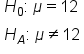The company took a random sample of 10 cans of its soda off the production line and got an average of 11.8 ounces with a standard deviation of 1.2 ounces.

We're looking at quantitative data, which is ounces of soda, and we also do not know the population standard deviation. Therefore, we're going to perform a t-test.

The first step is to calculate the t-statistic:The t-test statistic is negative 0.527. This score is plotted on the t-distribution below.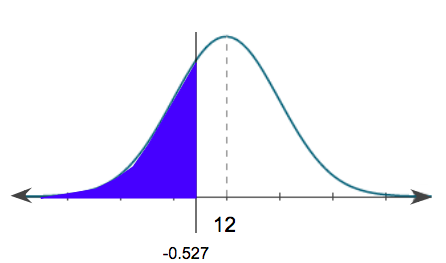2a. Graphing Calculator

Using a graphing calculator to go from the t-score to a p-value, we're going to look again at "tcdf." So go to 2nd, DIST, and we are going to scroll down to "tcdf," which stands for "t cumulative density function." We're going to put in the lower boundary of the shaded region, the upper boundary of the shaded region, then the degrees of freedom.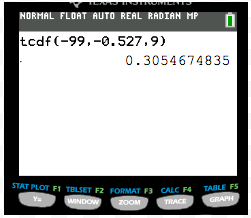The lower boundary of the shaded region for this problem is negative infinity. In order for the calculator to recognize negative infinity, we put in a negative 99. The upper boundary of the shaded region is the t-score, which is a negative 0.527. We have nine degrees of freedom, because our sample size was 10. We get a corresponding p-value of 0.3055, or 30.55%.

However, this is not our final answer. This was a two-sided test, and this is only the p-value that's associated with the left-tail, or the left-shaded region. We also have to get the corresponding upper-tail as well, which would fall at a positive 0.527.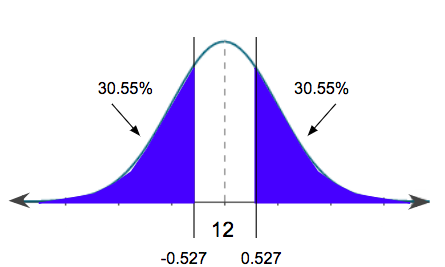Luckily, because these two areas are equal, all we have to do is take this p-value that we got on the calculator, and multiply it by two. The p-value for this problem is 0.3055 times 2, or 61.1%.

2b. T-Table

Now, let's find the p-value using the t-table. To get the p-value from the t-test statistic of a negative 0.527, remember, we're going to look at the corresponding degrees of freedom, which in this case was a nine, and we're going to find the closest t-score we can to what we calculated. The closest value to 0.527 is this 0.703.

t-Distribution Critical Values
Tail Probability, p
One-tail 0.25 0.20 0.15 0.10 0.05 0.025 0.02 0.01 0.005 0.0025 0.001 0.0005
Two-tail 0.50 0.40 0.30 0.20 0.10 0.05 0.04 0.02 0.01 0.005 0.002 0.001
df
1 1.000 1.376 1.963 3.078 6.314 12.71 15.89 31.82 63.66 127.3 318.3 636.6
2 0.816 1.080 1.386 1.886 2.920 4.303 4.849 6.965 9.925 14.09 22.33 31.60
3 0.765 0.978 1.250 1.638 2.353 3.182 3.482 4.541 5.841 7.453 10.21 12.92
4 0.741 0.941 1.190 1.533 2.132 2.776 2.999 3.747 4.604 5.598 7.173 8.610
5 0.727 0.920 1.156 1.476 2.015 2.571 2.757 3.365 4.032 4.773 5.893 6.869
6 0.718 0.906 1.134 1.440 1.943 2.447 2.612 3.143 3.707 4.317 5.208 5.959
7 0.711 0.896 1.119 1.415 1.895 2.365 2.517 2.998 3.499 4.029 4.785 5.408
8 0.706 0.889 1.108 1.397 1.860 2.306 2.449 2.896 3.355 3.833 4.501 5.041
9 0.703 0.883 1.100 1.383 1.833 2.262 2.398 2.821 3.250 3.690 4.297 4.781
10 0.700 0.879 1.093 1.372 1.812 2.228 2.359 2.764 3.169 3.581 4.144 4.587

There's nothing that we can estimate. We can't take an estimate between two values, because our t-score falls below the very first value. Because we're doing a two-sided test, we can just look at the row for two-tailed and get a p-value of 0.5, or 50%. We could have also looked at the one-tailed row and doubled the value of 0.25 to get the same answer.

2c. Excel

To convert our t-score into a p-value using Excel, we're going to go under the Formulas tab and insert a formula again under the Statistical column. But because this is a two-sided test, we want t-distribution dot 2t, or T.DIST.2T, for two tails. Even though the t-square that we calculated was negative 0.527, in Excel you always put in the positive tail. We're going to go ahead and put in the positive 0.527 with our degrees of freedom of 9.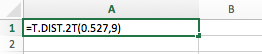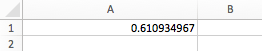Notice how we get the same p-value value of 61.1% that we did when using our calculator.

summary
Today we learned how to find a p-value from a t-test statistic using the calculator, the t-table table, and Excel. We did this for two types of test: a right-tailed test and a two-tailed test.

Good luck!

Source: Adapted from Sophia tutorial by RACHEL ORR-DEPNER.

of

## T-Table

of
Terms to Know
P-value

The probability that the test statistic is that value or more extreme in the direction of the alternative hypothesis

Test Statistic

A measurement, in standardized units, of how far a sample statistic is from the assumed parameter if the null hypothesis is true

Rating